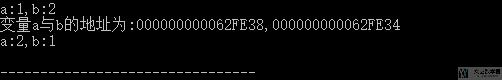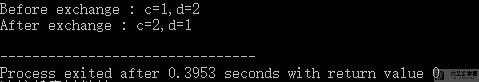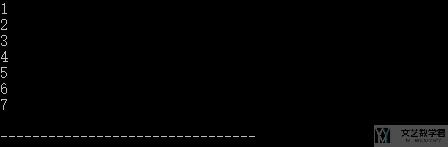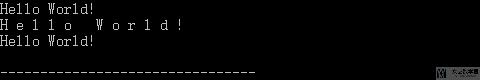# C学习笔记 – 指针

• 2
• 70 views
• A+

## 内容简介

• 指针的概念及定义：说明，指针变量的类型是指指针所指变量的类型，如 char *point是指point指向的是一个char类型的变量；
• 指针变量作为函数参数：举例，交换两个变量的值
• 通过指针操作数组；
• 通过指针引用字符串；

## 举例说明

### 指针的概念

1. #include<stdio.h>
2. #include<stdlib.h>
3. /*
4.     指针的概念 :
5.     - 指针里存放的是另一个变量的地址; int *p; p=&a;
6.     - 指针变量的类型是指 指针所指变量的类型(知道起始地址还要知道总长度)
7. */
8. int main(){
9.     int a=1,b=2,c;
10.     int *point_1,*point_2; //定义指向整数类型的指针变量,这里的*只是表示该变量类型是指针
11.     printf("a:%d,b:%d\n",a,b);
12.     point_1=&a; //指针point_1指向变量a的地址
13.     point_2=&b;
14.     printf("变量a与b的地址为:%p,%p\n",point_1,point_2); // %p为point的缩写,打印十六进制整数
15.     c = *point_1;
16.     a = *point_2;
17.     b = c;
18.     printf("a:%d,b:%d\n",*point_1,*point_2); // *point_1表示指针所指地址的值
19.     return 0;
20. }### 指针变量作为函数参数

1. #include<stdio.h>
2. #include<stdlib.h>
3. /*
4.     指针变量作为函数参数
5.     - 举例：交换两个变量的值
6. */
7. void swap(int *a,int *b); // 函数的声明
8. void swap(int *a,int *b){
9.     int temp;
10.     temp = *a;
11.     *a = *b;
12.     *b = temp;
13. }
14. int main(){
15.     int c=1,d=2;
16.     printf("Before exchange : c=%d,d=%d\n",c,d) ;
17.     swap(&c,&d);
18.     printf("After exchange : c=%d,d=%d\n",c,d) ;
19.     return 0;
20. }### 针对数组进行操作

1. #include<stdio.h>
2. #include<stdlib.h>
3. /*
4.     通过指针对数组进行操作
5.
6. */
7. int main(){
8.     int a={1,2,3,4,5,6,7};
9.     int *p;
10.     p = a; // 与 p=&a; 等价; 数组名代表数组首元素地址
11.     for(p=a;p<(a+7);p++){
12.         // 这里的p=p+1不是单纯的+1,而是因为指针指向int类型,有4字节, 故p+1是在内存中增加4个字节
13.         printf("%d\n",*p);
14.     }
15.     return 0;
16. }### 指针引用字符串

1. #include<stdio.h>
2. #include<stdlib.h>
3. /*
4.     本篇知识点
5.     - 通过指针引用字符串
6.     - 通过指针实现字符串复制
7. */
8. void string_copy(char *string_1,char *string_2); // 函数的声明
9. void string_copy(char *string_1,char *string_2){
10.     for(;*string_1!='\0';string_1++,string_2++){
11.         printf("%c ",*string_1);
12.         *string_2=*string_1;
13.     }
14.     *string_2='\0'; // 要在末尾加上结束的标志
15. }
16. int main(){
17.     char *p="Hello World!";
18.     char *q=malloc(15); //分配空间
19.     printf("%s\n",p);
20.     // 在输出项中给出字符指针变量名 string, 则系统会输出 string 所指向的字符串第一个字符, 然后自动使 string 加 1, 使之指向下一个字符，再输出该字符;
21.     // 如此直到遇到字符串结束标志 '\0', 因此再输出是能确定输出的字符到何时结束;
22.     // 错误写法 printf("%s\n",*p);
23.     // 使用指针实现字符串的复制
24.     string_copy(p,q);
25.     printf("\n%s\n",q);
26.     free(q);
27.     return 0;
28. }• 微信公众号
• 关注微信公众号
•• QQ群
• 我们的QQ群号
•## 目前评论：2   其中：访客  0   博主  0

•梦一帆

我也写了一篇
但是没这么详细https://www.fivk.cn/archives/123.html

•王 茂南 Admin

@梦一帆 写的非常好呀，一起相互学习。